Secure Site Shop with Confidence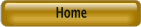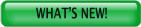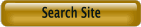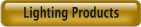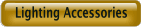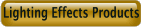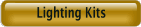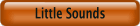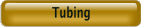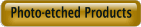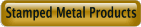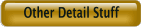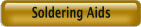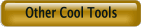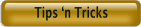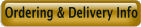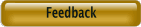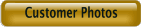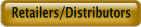Best viewed using:  Internet Explorer or Mozilla Firefox Intelligent LED Tester   Red (+) and Black (-) spring-loaded test clips to hold LEDs with wires or leads. Clips made of nickel-silver for excellent conductivity and durability. State-of-the-art current source component regulates LED current to within 2% High-strength .062" thick FR4 circuit board makes tester nearly indestructible.   Experimenting with variations in LED brightness   If you wish to use the tester to experiment with varying LED brightness (lowering current through the LED to reduce intensity), simply hold a surface-mount resistor over the two plated-through holes on the tester (marked R1) so each end of the resistor contacts one of the holes, or slide the leads of an axial resistor into the holes. Since this tester uses a constant current source that is independent of a fixed supply voltage to power the LED under test, a shortened version of the standard formula is used. To calculate the resistor value, divide the LED device voltage by the milliamps (ma) you wish to reduce. This will give the value in ohms of the resistor. Example: Reduce the current output from 20 ma to 10 ma with a red LED having a device voltage of 1.7. Divide 1.7 by 0.010 (10 ma). The result equals 170. Use a 170 ohm resistor.   Important note: The formula used here is: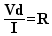where Vd is voltage of the device (LED), I is current and R is resistor that is calculated. This formula should only be used for calculations for resistors connected to the constant current source on this LED tester. This formula does not include the variable (Vs) for a voltage source (power supply) that is part of the standard formula used to calculate resistors for most LED circuits. This standard formula is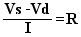and a complete explanation with circuits using it, is here.   © 2008 Ngineering